error: Content is protected !!

# PHYSICS: FORM TWO: Topic 6 - MOTION IN STRAIGHT LINE

TOPIC 6: MOTION IN STRAIGHT LINE
Motion is the change of position of an object from one place to another. It is the study of the movement of a body from one point to another. It studies of all aspects of motion including distance and displacement, speed and velocity, acceleration and deceleration e.t. c.
There are two types of motions;
1. Circular motion- Is the motion of an object in a circular path. Examples; Motion of the electron around the nucleus of an atom, Revolutionary movement of the earth around the sun, Motion of a car around a cornered road.
2. Linear motion- Is the motion of an object in a straight-line path. Examples; A fruit falling from a tree, a stone thrown vertically upward, a bus moving along a straight road.
Difference between Distance and Displacement
Distinguish between Distance and Displacement
Distance is the length between two points or two places. It is a length covered by a body in motion. Distance is a scalar quantity because it has magnitude only. For example, a car moved 4 km. ‘4 km’ is the magnitude of the distance covered.
Displacement is the length between two points in a specific direction. It is simply a distance covered by a moving body in a specific direction. Displacement is a vector quantity because it has magnitude and direction. For example, a car moving through 4 km towards north. ‘4 km’ is the magnitude of the displacement and ‘north’ is the direction of the motion of the car.
Differences between distance and displacement
 Distance Displacement Is the length moved by an object between two points Is the distance in a specific direction It is a scalar quantity It is a vector quantity

The SI Units of Distance and Displacement
State the SI units of Distance and Displacement
Distance and displacement have the same S.I unit which is Metre (m). other common units used to measure distance and displacement are Kilometre (km), centimetre (cm), millimetre (mm), miles and so on.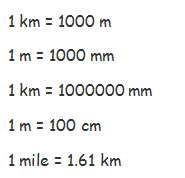Difference between Speed and Velocity
Distinguish between Speed and Velocity
Speed is the distance moved by an object per second. It is the rate of change of distance. It is obtained by dividing the distance moved by the objects to the time taken. Speed is a scalar quantity hence have magnitude only.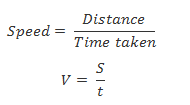Velocity is the displacement per second. It is simply the speed of a body in a specific direction or the rate of change of displacement. Velocity is obtained by dividing the displacement to the time taken. Velocity is a vector quantity.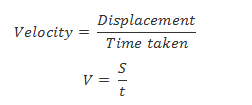The SI Unit of Speed and Velocity
State the SI unit of Speed and Velocity
The SI unit of speed and velocity the same namely metre per second (m/s). The other common unit are Kilometre per hour (km/h).
Conversion between km/h and m/s.
To change 1 km/h to m/s;
1 kilometre is changed into metre by multiplying it with 1000 while 1 hour is changed into seconds by multiplying with 60 x 60 as shown in the equation below.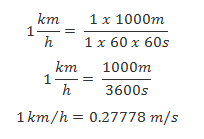To change 1 m/s to km/h;
1 m is changed into km by dividing it by 1000 while 1 sec is changed to hours by dividing it by 60 x 60 as shown below.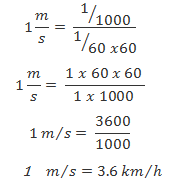The Average Velocity of a Body
Determine average velocity of a body
Average velocity is the average or mean value between initial velocity (U) and final velocity (V). It is also defined as the ratio of the total displacement to the total time.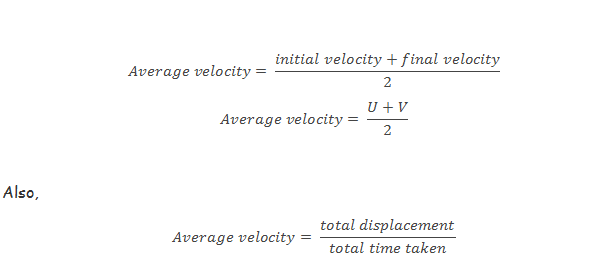The following are important terminologies related to velocity of a moving body;
1. Initial velocity (U)– is the velocity of a body at the start of observation.
2. Final velocity (V)– is the velocity of a body at the end of observation
3. Uniform or constant velocity- Is the velocity where by the rate of change of displacement with time is constant.
4. Absolute velocity- is the actual velocity of a moving object recorded by a stationary observer
5. Relative velocity- is the velocity of a moving object recorded by a moving observer.
6. Instantaneous velocity- is the velocity of a moving object recorded at any time.

Acceleration is the rate of change of velocity. It is the change in velocity per unit time. It is obtained by dividing the change in velocity of a moving body to the time taken. Acceleration is a vector quantity.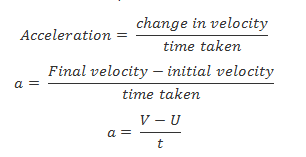Velocity Time-graph
Interpret velocity time-graph
This is a graph drawn to represent motion of a body. Velocity is represented by the vertical axis while time is represented by its horizontal axis. Consider a body accelerating uniformly from rest to a certain velocity v within time t. This can be represented graphically as shown below;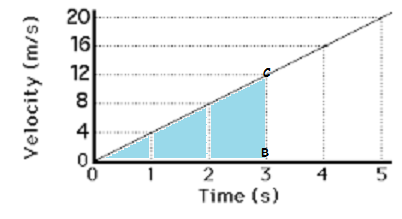In the above graph, the body attained a velocity of 8m/s within 2 seconds. It also attained 16m/s within 4 seconds. It reached its final velocity (v) 20m/s at 5 seconds. The initial velocity (u) was 0m/s.
Distance travelled
The distance covered by a body can be calculated from a velocity-time graph by calculating the area covered under the curve/graph. In this case the area under the velocity-time graph is always the area of the triangle.
Consider the shaded area (triangle OBC) on the above graph;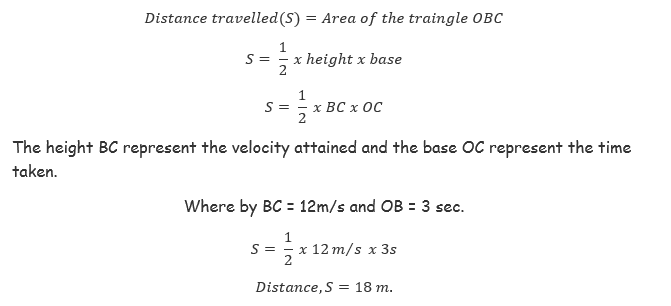Acceleration
Acceleration can be calculated from the graph by calculating the slope of the graph. Slope of the velocity-time graph is obtained as the ratio of the change in velocity to a respective change in time.Consider again the shaded region of our previous graph in where by velocity changed from u =0m/s to v =12m/s along vertical axis BC and the time changed from t=0s to t2 =3s along horizontal axis OB.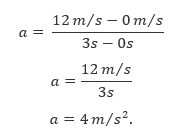Therefore, the body accelerated by 3m/s2 by changing its velocity from 0m/s to 12m/s within 3s.

The Acceleration of a Body
Determine the acceleration of a body
A body is a said to accelerate when its velocity is increasing with time. That is, the final velocity v, is always smaller to the initial velocity u. See the below examples;
Example 1
A car starts from rest and accelerates to a velocity of 120m/s in one minute. Calculate the acceleration of the car.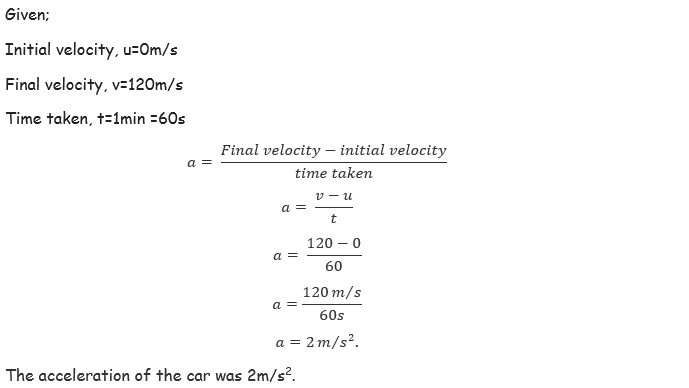Example 2
A train accelerates from 40m/s to 110m/s. What is the acceleration of the train if it changes the velocity in 14 seconds.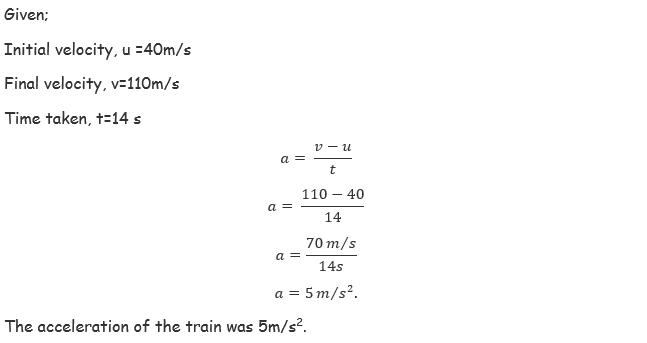The Concept of Retardation
Explain the concept of retardation
Retardation or deceleration is simply the opposite of acceleration. It is the negative acceleration. Retardation is defined as the rate of decrease of velocity. It is the decrease in velocity per unit time.
A body in motion can accelerate, decelerate or move with uniform acceleration. Uniform acceleration is when a body is having constant rate of increase in velocity.
Note;
• When a body starts from rest or is brought to rest, its velocity is zero.
• When the velocity of a body is constant or uniform, its acceleration is zero.
• When the velocity of a moving object increases, its acceleration becomes positive.
• When the velocity of a moving object decreases, its acceleration becomes negative called retardation.

Equation of Uniformly Accelerated Motion
Derive equation of uniformly accelerated motion
The motion of a body in a straight line can be described by using three equations known as equations of motion.
First equation of motion;
Consider a body initially moving with initial velocity ‘u’ and after a certain time ‘t’ it accelerates to a final velocity ‘v’. For that case,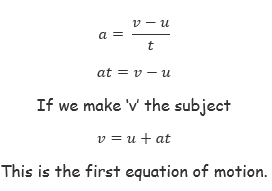Second equation of motion;
Consider a body moving with uniform acceleration. Its average velocity can be obtained as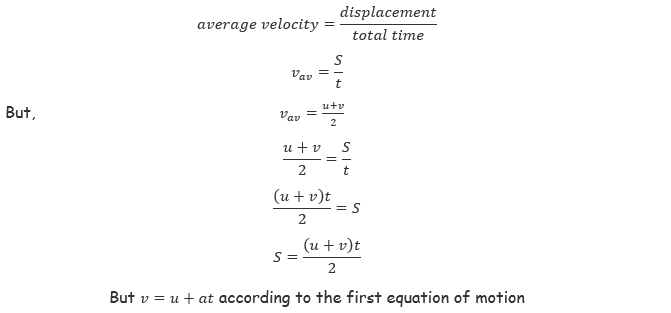Therefore,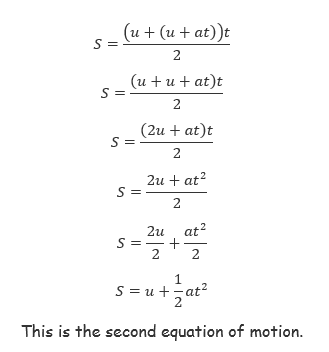Third equation of motion;
The third equation of motion can be obtained by squaring the first equation on both sides.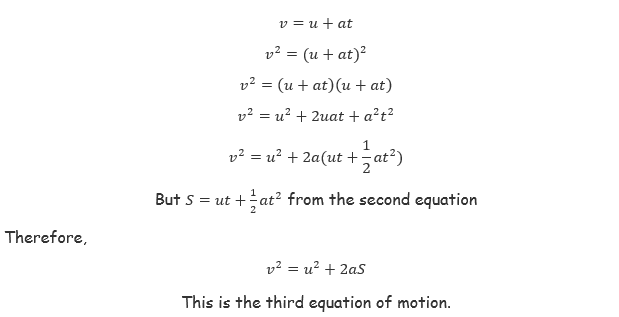Equations of Accelerated Motion in Daily Life
Apply equations of accelerated motion in daily life
The three equations can be used to solve many problems regarding the motion of different bodies.
Example 3
An object moves at 20m/s due north at an acceleration of 2m/s2. What will be its final velocity after it has moved 21 m?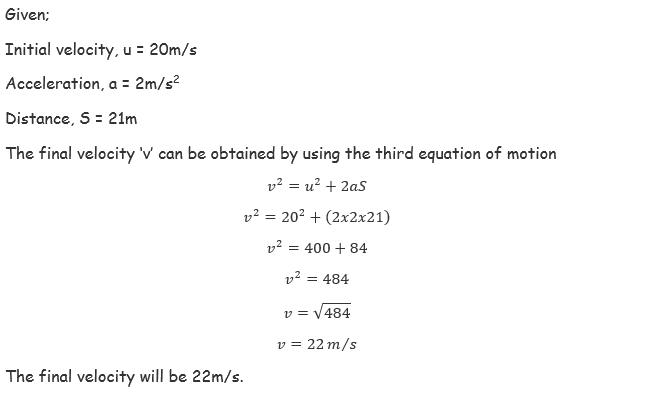Example 4
An object travelling at 12m/s accelerates at 4m/s2 for 16 s.
• Calculate the final velocity
• How far does it travel for 16 seconds?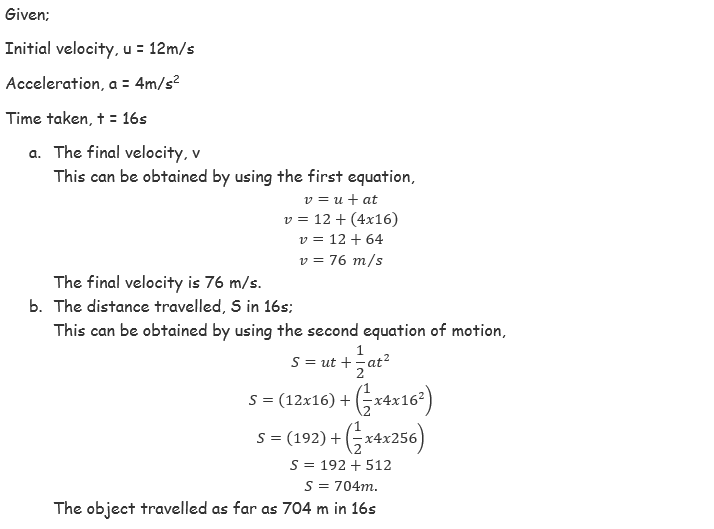The Concept of Gravitational Force
Explain the concept of gravitational force
The acceleration of a free falling body is known as the acceleration due to gravity denoted by g and controlled by gravitational force of the earth.
When two bodies of different masses are released from a certain height h above the ground, they will reach the ground at different times with the heavier one reaching earlier before the lighter one.
The reason is that the air resistance is more effective on lighter bodies than in heavier bodies, consequently this affect acceleration due to gravity in a reverse manner.
But dropping a light object and a heavy object in a vacuum they will reach the ground at the same time due to the absence of air resistance effect.
Gravitational force affects the motion of a body positively or negatively. A body released freely from above the earth's surface is positively affected by gravitational force. This is because it is moving towards the earth. On the other side, the body thrown away from the earth's surface is affected negatively by the gravitational force. This is because it is moving against the earth's gravitational pull. These two kinds of motion can be described using the three equations of motion.
Acceleration due to Gravity
Determine acceleration due to gravity
The easiest way of determining the value for the acceleration of gravity is by observing the motion of a simple pendulum. This kind of experiment was first done by Galileo Galilei when he was studying the uniformly accelerated motion.
A simple pendulum is a small heavy body suspended by a light inextensible string from a fixed support and it is normally used to determine acceleration due to gravity.
It is made by attaching a a long thread to a spherical ball called a pendulum bob. The string is held at a fixed at a fixed support like two pieces of wood held by a clamp and stand.
If the bob is slightly displaced to position B,it swings to and from going to C through O and back to B through O. When the pendulum complete one cycle/revolution the time taken is called the period of oscillation, T.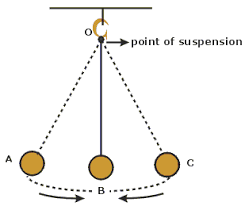Definitions
1. Period ,T is the time taken by the pendulum bob to complete one complete cycle.
2. Angular displacement is the angle made between the string and the vertical axis when the bob is displaced to a maximum displacement.
3. Amplitude is the maximum displacement by which the pendulum has been displaced.
4. Length of pendulum is the length of the string from the point of attachment on the wooden pieces to the canterof gravity of the bob.
From the experiments, it has been observed that, changing the weight of the bob and keeping the same length of pendulum, the period is always constant provided that all swings are small though they may be different in size.
The period T of the pendulum is given by;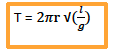Where; l = length of pendulum; g = acceleration due to gravity
Also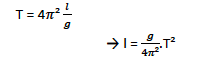It follows that if we plot a graph of l against T. it is going to be a straight line with a slope g/4Π2and y –intercept equal to 0.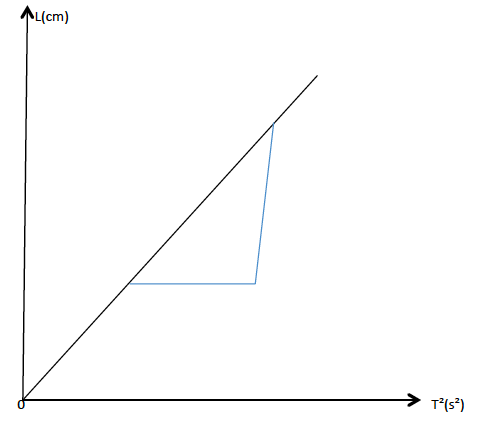When the bob is raised to point B it will gain potential energy and the bob will swing due to the conservation of energy from potential to kinetic energy.
• At B and C all energy is P.E.
• At O all energy is K.E.
• P.E at B = K.E at O.
If the pendulum swung in vacuum the oscillations would have been continuous. But practically, air friction causes losses of energy of the pendulum bob. That is why after a certain time the oscillations cease.

The Application of Gravitational Force
Explain the application of gravitational force
Gravitational force is applied in many events of our daily life;
• Aid organizations and military aircraft use the gravitational force to supply food parcels and other useful parcels to the needing individuals.
• It is used by parachutist when they free-fall from the sky.
• We easily get fruits from trees as they freely fall to the ground after have been detached from the branches.
• Astronauts can plan well their journey to the space by using the values of the gravitational force around heavenly objects in the universe.
Activity
Experiment
Aim: Determination of acceleration due to gravity by using the simple pendulum.
Materials and apparatus: A simple pendulum, stand, clamps and stop watch.
Procedures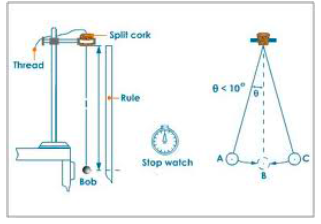• Suspend a bob with a thread between wooden pieces by clamping them on to a stand.
• Pull the bob slightly to one side and release the bob. Make sure the bob swings 50 complete oscillations.
• To be more accurate, perform three measurements for each length l of the pendulum.
• Repeat the procedures with l = 60cm and 50cm.
• Record the results as in the table below. -Plot the graph of l against T2
Results
 Length, l (cm) Time for 50 oscillations. T (s) T² (s²) t₁ (s) t₂ (s) t₃ (s) Average(s)
Observation
The graph obtained will be a straight line through the origin.
The slope of the graph is given by;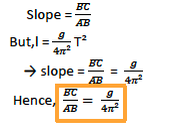From this equation, the acceleration due to gravity can be computed easily.

#### 1 comment:

1.Good notes but you can add more questions.